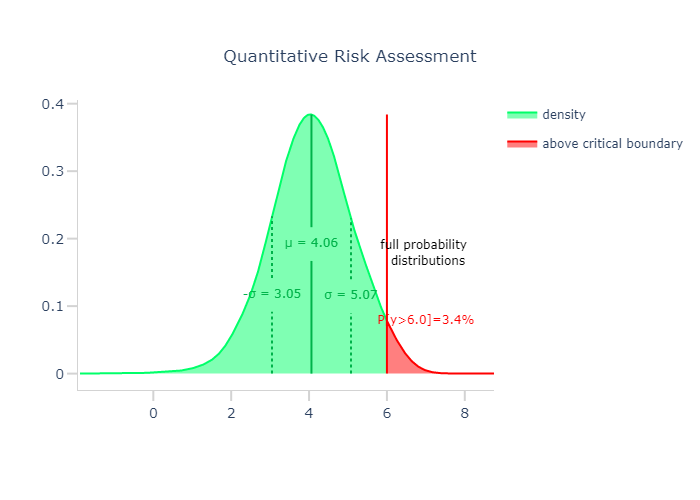# Full densities instead of point predictions#

:

import numpy as np
import halerium.core as hal
from IPython.display import Image

g = hal.Graph("g")
with g:
x = hal.Variable("x")

y = hal.Variable("y")
y.mean = x * 3. + 1.
y.variance = 1.

# can be used to visualize the graph on the Halerium platform
#hal.show(g)

x_data = [1.]

model = hal.get_generative_model(g, dl)
prediction_samples = model.get_samples(g.y, n_samples=1000)
prediction_samples = np.array(prediction_samples)[:,0]


## What is the uncertainty estimate good for?#

Imaging there is a hard limit at a output value of 6. Then you have to know what is the likelihood of the real value actually being over 6.

:

boundary=6.


### Plotly Visualization#

:

from plots import plot_density_with_boundary

Image(plot_density_with_boundary(prediction_samples, boundary, smoothness=10.))

:[ ]: